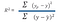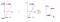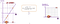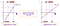# Regression Models

Regression is a technique that displays the relationship between variable y based on values of x

ex: y- Inches of rain varies according to x- New Cars sold

• If you think there is a relationship between two things, the regression would help to confirm it.

Main Types:

Linear Regression — Continuous Variables, Solves Regression Issue, Straight Line

Logistic Regression- Categorical Variables, Solves Classification Issue, s curve

• plotting a line equation, Y=mx+C
• Simple Linear regression is useful for finding the relationship between two continuous variables. One is the independent variable, and the other is the dependent variable
• Good for a problem involving finding the exact value of Y for given X value. Like finding House Size(y) for given Money(x) but not suited for Classification problems like if the house is in a good locality or not.

Video Explaining Step by Step Linear Regression

Least Square Error — the process used to plot the regression line

steps: calculate mean of x and y, the regression line will always pass through mean of collective x and y point values.

Then find value of m and c using mean point in eqn y=mx+c, then find best fit then R2 value.

R2 value — Goodness of Fit Rotation of Regression line https://www.youtube.com/watch? (tell if independent variable is dependent on dependent variable or not and by how much)higher R2 means error is less between predicted to actual point also indicates highly correlated

Standard Error of Estimate

Basic Linear regression in Python — Plotting and y=b0+b1x coefficients

`import numpy as npimport matplotlib.pyplot as pltdef estimate_coefficient(x,y):    n=np.size(x)    mean_x,mean_y=np.mean(x),np.mean(y)    SS_xy = np.sum(y*x - n*mean_y*mean_x)    SS_xx = np.sum(x*x - n*mean_x*mean_x)    b1=SS_xy/SS_xx    b0=mean_y-b1*mean_x    return(b0,b1)def plot_regression_line(x,y,b):    plt.scatter(x,y,color='m',marker="o")    y_pred=b+b*x    plt.plot(x,y_pred,color='g')    plt.xlabel('Size')    plt.ylabel('Cost')    plt.show()x=np.array([1,2,3,4,5,6,7,8,9,10])y=np.array([300,350,500,700,800,850,900,900,1000,1200])b=estimate_coefficient(x,y)print("estimated Coefficients :\nb0 = {} \nb1 = {}".format(b,b))plot_regression_line(x,y,b)`

Logistic Regression

• A statistical classification model
• Deals with categorical dependent variables
• could be binary or distinct values, multinominal
• Takes both continuous and discrete input data
• Gives outcome in terms of probability, which helps in classifying
• works well with the large volume of the dataset

Example: Basic Spam Email classifier

1. Define the variables

Independent variables — count of spam words

ex of spam words: Lottery, Winner, Crores, Free, etc

Dependent variable — label Spam(1) and Not Spam(0)

2. Plot Labeled data

3. Draw Regression Line

Steps of creating Sigmoid curve for the best fit

1. convert probability of the scale of 0 to 1 to scale of log(odds) that range from +ve infinity to -ve infinity

Probability = Favourable Events /Total events

Odds= Favourable Events/Unfavourable events

Log(odds) =Logit Function

Log(odds ratio) = Log(odds for case1/odds for case2)

For our spam classifier case, converting scale of Probability 0,1 to scale of log(odds)convert to the scale of log(odds) then Finally convert to Sigmoid Curve
`>>> To convert scale of probability between 0 and 1 to more meaningful y axis graph , we convert it to log(odds) using formula =log(p/1-p). Line passing through zero corresponds p=.5log(odds) = log(P(spam)/1-P(spam))= log(1/1–1) =log(1/0) -> + ∞log(odds) = log(P(Not Spam)/1-p(Not Spam) =log(0/1) -> -∞`
• Sigmoid Curve helps in classification problems as in Fig, Ye value is predicted based on Xe value. Since Ye>0.5 we can say it will be classified as label 1.
• can take any real value as input and map to a value between 0 to 1

4. Find the best Fit using MLEFirst — Calculate Probability from log(odds) scale of each data point second- Find likelihood for each data point using the above equation then sum up all log of all likelihood to get the measure of Fit. Above Eqn is used to convert log(odds) to sigmoid curveThird- Rotate the line and find log(likelihood) of lines, we would get a measure to find a better fit

Regression Models using sklearn Package

Using Boston DataSet, Another Hello World program for Linear regression problem

Dataset:

.